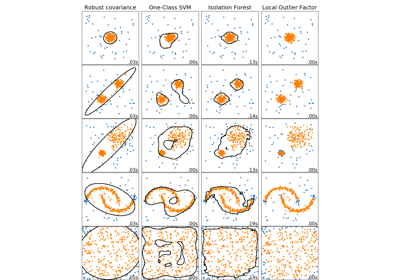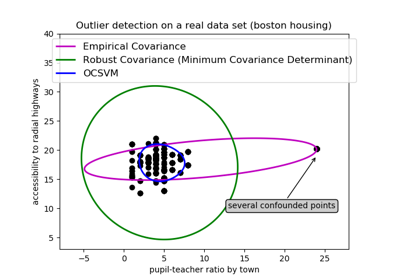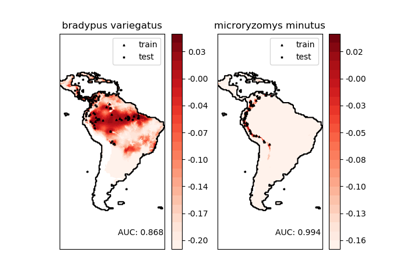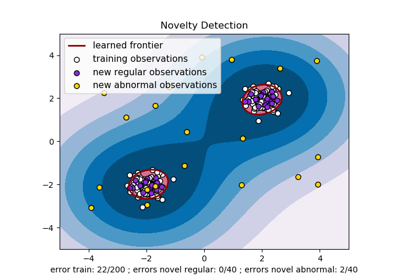# sklearn.svm.OneClassSVM¶

class sklearn.svm.OneClassSVM(kernel='rbf', degree=3, gamma='scale', coef0=0.0, tol=0.001, nu=0.5, shrinking=True, cache_size=200, verbose=False, max_iter=-1)[source]

Unsupervised Outlier Detection.

Estimate the support of a high-dimensional distribution.

The implementation is based on libsvm.

Read more in the User Guide.

Parameters
kernelstring, optional (default=’rbf’)

Specifies the kernel type to be used in the algorithm. It must be one of ‘linear’, ‘poly’, ‘rbf’, ‘sigmoid’, ‘precomputed’ or a callable. If none is given, ‘rbf’ will be used. If a callable is given it is used to precompute the kernel matrix.

degreeint, optional (default=3)

Degree of the polynomial kernel function (‘poly’). Ignored by all other kernels.

gamma{‘scale’, ‘auto’} or float, optional (default=’scale’)

Kernel coefficient for ‘rbf’, ‘poly’ and ‘sigmoid’.

• if gamma='scale' (default) is passed then it uses 1 / (n_features * X.var()) as value of gamma,

• if ‘auto’, uses 1 / n_features.

Changed in version 0.22: The default value of gamma changed from ‘auto’ to ‘scale’.

coef0float, optional (default=0.0)

Independent term in kernel function. It is only significant in ‘poly’ and ‘sigmoid’.

tolfloat, optional

Tolerance for stopping criterion.

nufloat, optional

An upper bound on the fraction of training errors and a lower bound of the fraction of support vectors. Should be in the interval (0, 1]. By default 0.5 will be taken.

shrinkingboolean, optional

Whether to use the shrinking heuristic.

cache_sizefloat, optional

Specify the size of the kernel cache (in MB).

verbosebool, default: False

Enable verbose output. Note that this setting takes advantage of a per-process runtime setting in libsvm that, if enabled, may not work properly in a multithreaded context.

max_iterint, optional (default=-1)

Hard limit on iterations within solver, or -1 for no limit.

Attributes
support_array-like of shape (n_SV)

Indices of support vectors.

support_vectors_array-like of shape (n_SV, n_features)

Support vectors.

dual_coef_array, shape = [1, n_SV]

Coefficients of the support vectors in the decision function.

coef_array, shape = [1, n_features]

Weights assigned to the features (coefficients in the primal problem). This is only available in the case of a linear kernel.

coef_ is readonly property derived from dual_coef_ and support_vectors_

intercept_array, shape = [1,]

Constant in the decision function.

offset_float

Offset used to define the decision function from the raw scores. We have the relation: decision_function = score_samples - offset_. The offset is the opposite of intercept_ and is provided for consistency with other outlier detection algorithms.

fit_status_int

0 if correctly fitted, 1 otherwise (will raise warning)

Examples

>>> from sklearn.svm import OneClassSVM
>>> X = [, [0.44], [0.45], [0.46], ]
>>> clf = OneClassSVM(gamma='auto').fit(X)
>>> clf.predict(X)
array([-1,  1,  1,  1, -1])
>>> clf.score_samples(X)  # doctest: +ELLIPSIS
array([1.7798..., 2.0547..., 2.0556..., 2.0561..., 1.7332...])


Methods

 decision_function(self, X) Signed distance to the separating hyperplane. fit(self, X[, y, sample_weight]) Detects the soft boundary of the set of samples X. fit_predict(self, X[, y]) Perform fit on X and returns labels for X. get_params(self[, deep]) Get parameters for this estimator. predict(self, X) Perform classification on samples in X. score_samples(self, X) Raw scoring function of the samples. set_params(self, \*\*params) Set the parameters of this estimator.
__init__(self, kernel='rbf', degree=3, gamma='scale', coef0=0.0, tol=0.001, nu=0.5, shrinking=True, cache_size=200, verbose=False, max_iter=-1)[source]

Initialize self. See help(type(self)) for accurate signature.

decision_function(self, X)[source]

Signed distance to the separating hyperplane.

Signed distance is positive for an inlier and negative for an outlier.

Parameters
Xarray-like, shape (n_samples, n_features)
Returns
decarray-like, shape (n_samples,)

Returns the decision function of the samples.

fit(self, X, y=None, sample_weight=None, **params)[source]

Detects the soft boundary of the set of samples X.

Parameters
X{array-like, sparse matrix}, shape (n_samples, n_features)

Set of samples, where n_samples is the number of samples and n_features is the number of features.

sample_weightarray-like, shape (n_samples,)

Per-sample weights. Rescale C per sample. Higher weights force the classifier to put more emphasis on these points.

yIgnored

not used, present for API consistency by convention.

Returns
selfobject

Notes

If X is not a C-ordered contiguous array it is copied.

fit_predict(self, X, y=None)[source]

Perform fit on X and returns labels for X.

Returns -1 for outliers and 1 for inliers.

Parameters
Xndarray, shape (n_samples, n_features)

Input data.

yIgnored

Not used, present for API consistency by convention.

Returns
yndarray, shape (n_samples,)

1 for inliers, -1 for outliers.

get_params(self, deep=True)[source]

Get parameters for this estimator.

Parameters
deepbool, default=True

If True, will return the parameters for this estimator and contained subobjects that are estimators.

Returns
paramsmapping of string to any

Parameter names mapped to their values.

predict(self, X)[source]

Perform classification on samples in X.

For a one-class model, +1 or -1 is returned.

Parameters
X{array-like, sparse matrix}, shape (n_samples, n_features)

For kernel=”precomputed”, the expected shape of X is [n_samples_test, n_samples_train]

Returns
y_predarray, shape (n_samples,)

Class labels for samples in X.

score_samples(self, X)[source]

Raw scoring function of the samples.

Parameters
Xarray-like, shape (n_samples, n_features)
Returns
score_samplesarray-like, shape (n_samples,)

Returns the (unshifted) scoring function of the samples.

set_params(self, **params)[source]

Set the parameters of this estimator.

The method works on simple estimators as well as on nested objects (such as pipelines). The latter have parameters of the form <component>__<parameter> so that it’s possible to update each component of a nested object.

Parameters
**paramsdict

Estimator parameters.

Returns
selfobject

Estimator instance.

## Examples using sklearn.svm.OneClassSVM¶